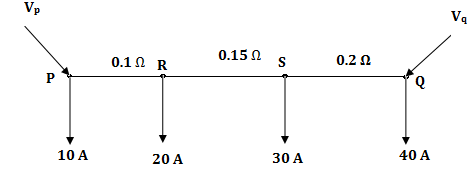# Numerical - Calculate voltage - Power System

Q.  A distribution system is shown in figure with load currents as marked. The two ends of the feeder are fed by voltage sources such that Vp = Vq = 3 V, the value of the voltage Vp for a minimum voltage of 220 V at any point along the feeder is- Published on 23 Nov 15

a. 225.89 V
b. 222.89 V
c. 220.0 V
d. 228.58 V